THE SHAFTS WERE VERY SUBSTANTIAL, LABOURIOUSLY EXCAVATED STRUCTURES.

Given the immense amount of effort undertaken by the ancient society to excavate just one shaft, let alone 20, it would be unsurprising to find that they were built for much more than one code-identifying saunter around the park. There was something far more sophisticated in their design layout, rendering the shaft positions multi-functional.

We'll now do another circuit, but this time only move between every second shaft position to see if clear cut distance and angle codes can still be extracted.

1A TO 2A.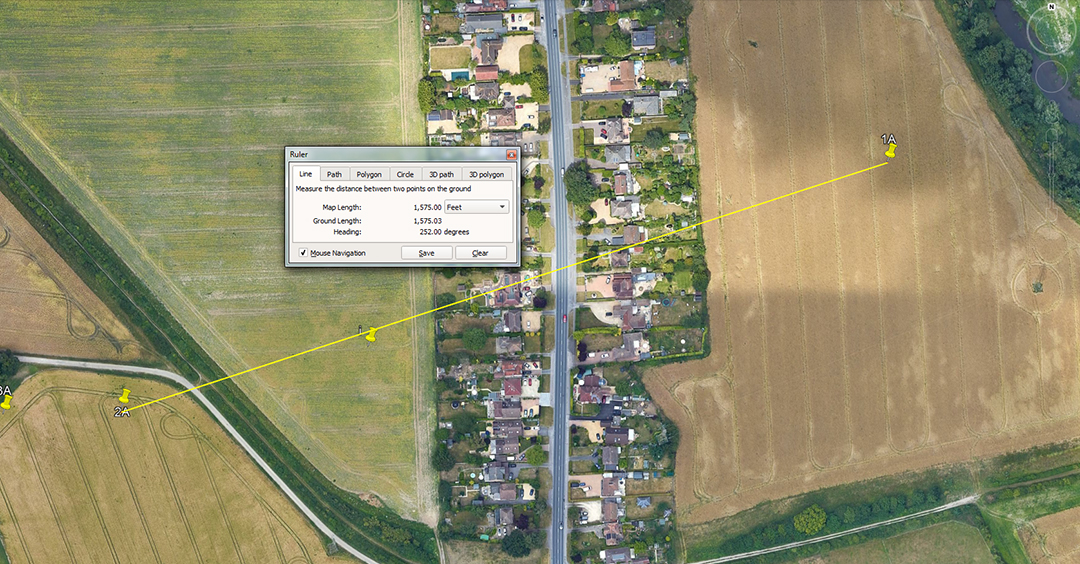The distance is 1575-feet and the angle is 252-degrees (reverse angle 72-degrees). The merits of 1584-feet would also be featured.

A mathematical progression based upon 1575 goes:

1575, 3150, 4725, 6300, 7875, 9450, 11025, 12600, 14175, 15750, 17325, 18900, 20475, 22050, 23625, 25200 ... 28350 ... 37800 ... 75600, etc.

We see in this string increments of length that relate to the mile of 5250-feet and an equatorial circumference of 130636800-feet. The values generated are also much used in lunar period calculations.

A mathematical progression based upon 1584-feet goes:

1584, 3168, 4752, 6336, 7920, 9504, 11088, 12672, 14256, 15840, 17424, 19008, 20592, 22176, 23760, 25344 ... 28512 ... 38016 ... 76032. etc.

We see in this string increments of length that relate to the mile of 5280-feet and an equatorial circumference of 130680000-feet.

The merits of the angle 252-degrees (reverse angle 72-degrees) has already been covered relative to shaft 11D to shaft 12D.

2A TO 4A.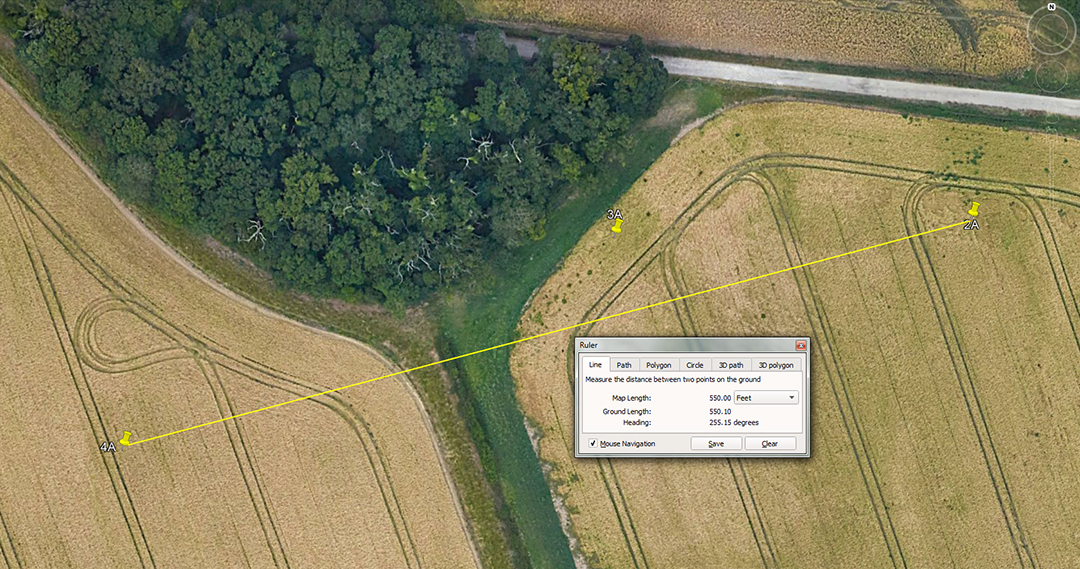The distance is 550-feet and the angle is 255.15-degrees. A tutorial about the merits of 255.675 would also have featured.

The codes related to 550-feet are covered in the section 6A to 7A.

The angle relates to a tutorial about the number of lunar days counted within the Sabbatical Calendar system (2551.5-days or 7.2 lunar years of 354.375-days duration).

A second tutorial would identify the number of solar days in 7 solar years, counted alongside the lunar period within the lunisolar Sabbatical Calendar system (2556.75-days @ 365.25-days per annum).

4A TO 6A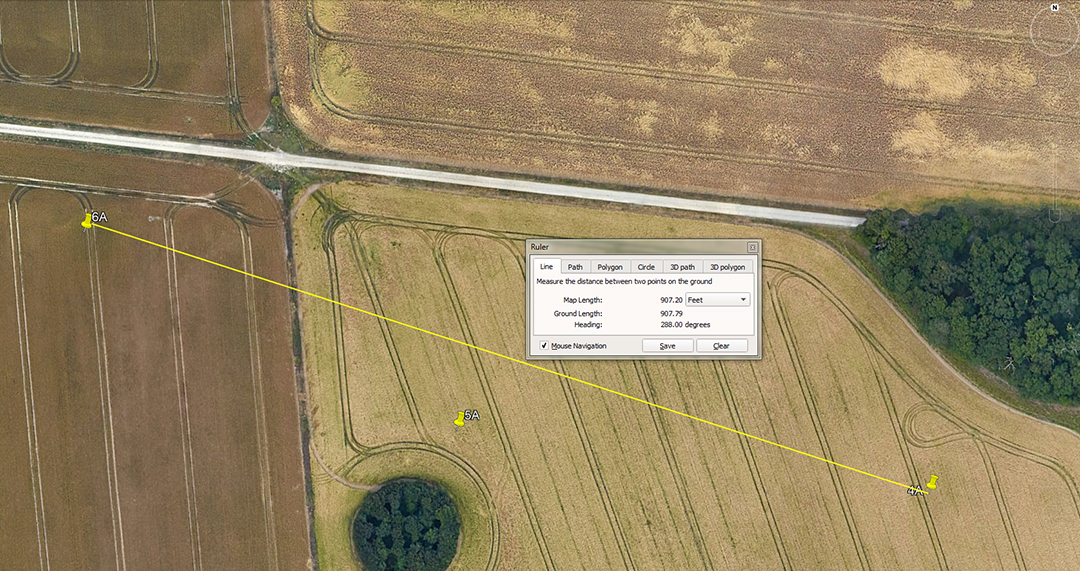The distance is 907.2-feet and the angle is 288-degrees. The value 907.5' would also have featured.

A mathematical progression based upon 9072 goes:

9072, 18144, 27216, 36288, 45360, 54432, 63504, 72576, 81648, 90720 ... 226800 ... 362880 ... 130636800.

The inch value for 1 side length of the Great Pyramid is 9072-inches (756-feet X 12) and the string advances to the number of feet used to describe the equatorial circumference of the Earth under the navigational system that used 12 X 12 X 12 X 12 X 1.2 Greek miles of 5250-feet each = 24883.2-miles. Within this reading, 1-degree of arc would equate to 362880-feet.

But the Great Pyramid's coding had to include yet another navigational method that used the slightly longer mile of 5280-feet. For this, the length of one side of the pyramid had to be viewed as representing 756.25-feet or 3-inches longer (9075-inches). A mathematical progression based upon 9075-inches goes:

9075, 18150, 27225, 36300, 45375, 54450, 63525, 72600, 81675, 90750 ... 226875 ... 363000 ... 130680000.

Under this method, the equatorial circumference was considered to be 24750-miles of 5280-feet, for a complete circuit around the world, representing 130680000-feet and 1-degree of arc being 363000-feet.

Note also: Whereas the vertical height of the truncated Great Pyramid was 453.6-feet to the top of the flat floor viewing platform (264 cubits of 20.61818182-inches) under the 5250-feet reading, it represented 453.75-feet under the 5280-mile reading (264 cubits of 20.625-inches). In either case, (read as 45360-feet or 45375-feet) the readings represented 1/2880th of the respective navigational systems to which they were assigned.

6A TO 8A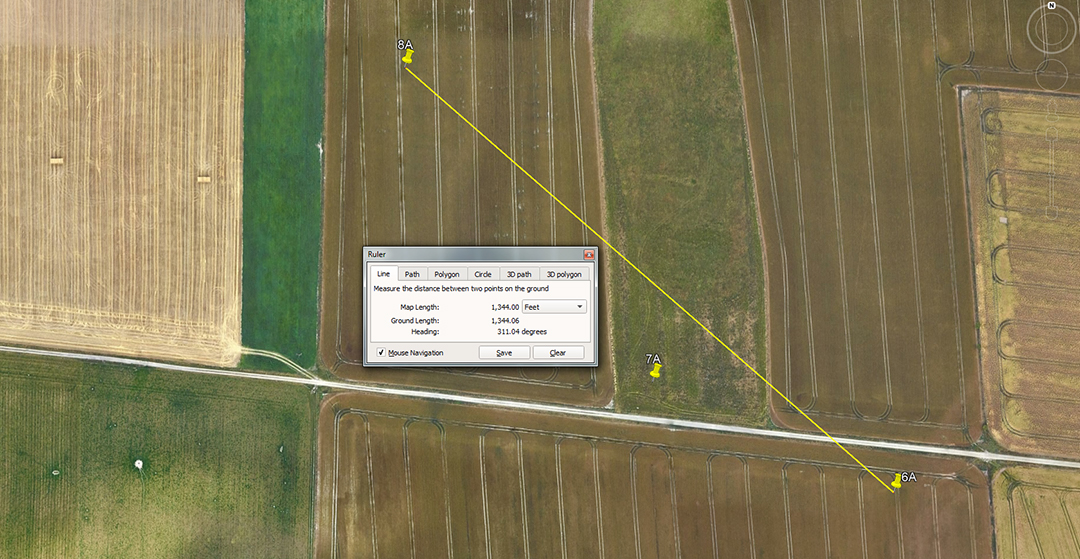The distance is 1344-feet and the angle is 311.04-degrees.

A mathematical progression based upon 1344 goes:

1344, 2688, 4032, 5376, 6720, 8064, 9408, 10752, 12096, 13440 ... 20160 ... 36288 ... 53760 ... 60480, etc.

Under this string, the sum of 1344-feet would be 1/270th of 1-degree of equatorial arc or 1/97200th of the full circumference of the Earth under the 24883.2 Greek mile (of 5250-feet) assignment.

The degree angle emphasises one of the most important values used in ancient navigation and 311.04-miles would be 1/80th of the 24883.2-mile equatorial circumference or 4.5-degrees of arc. See also the distance relationship between the epicentre of Silbury Hill and the southernmost obelisk at the entryway into West Kennet Longbarrow.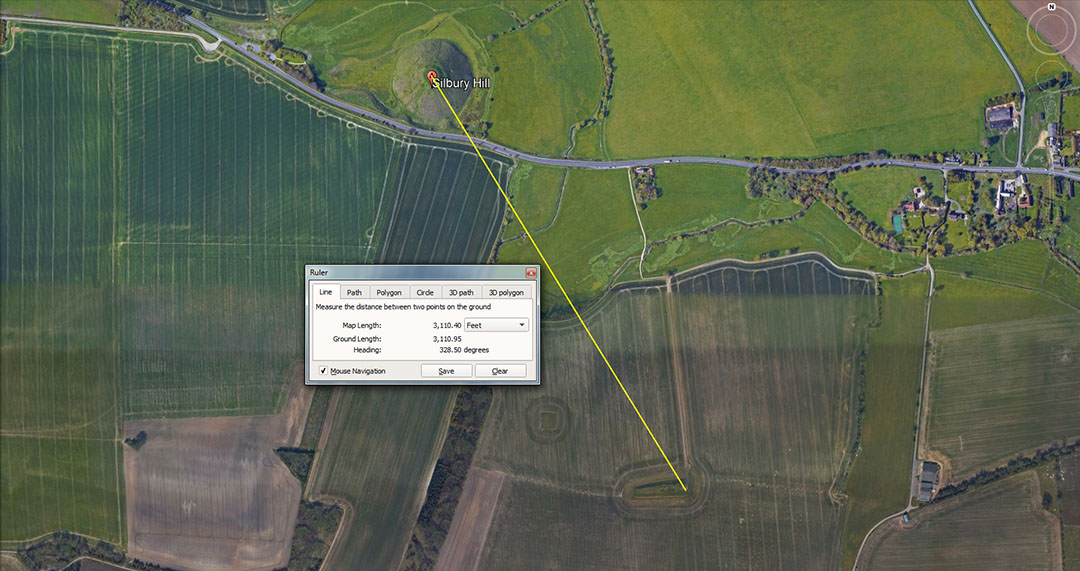From the centre of man-made, artificial, Silbury Hill near Avebury Henge, to West Kennet Longbarrow's southern-most entranceway alignment of obelisks, the distance is 3110.4-feet @ 148.5-degrees. This distance would be 1/42000th of the equatorial circumference of the Earth under the Great Pyramid's literal navigational assignment of 130636800-feet (24883.2-miles of 5250-feet). The sum of 3110.4-miles would be 1/8th of the 24883.2-mile circumference.

8A TO CURSUS EAST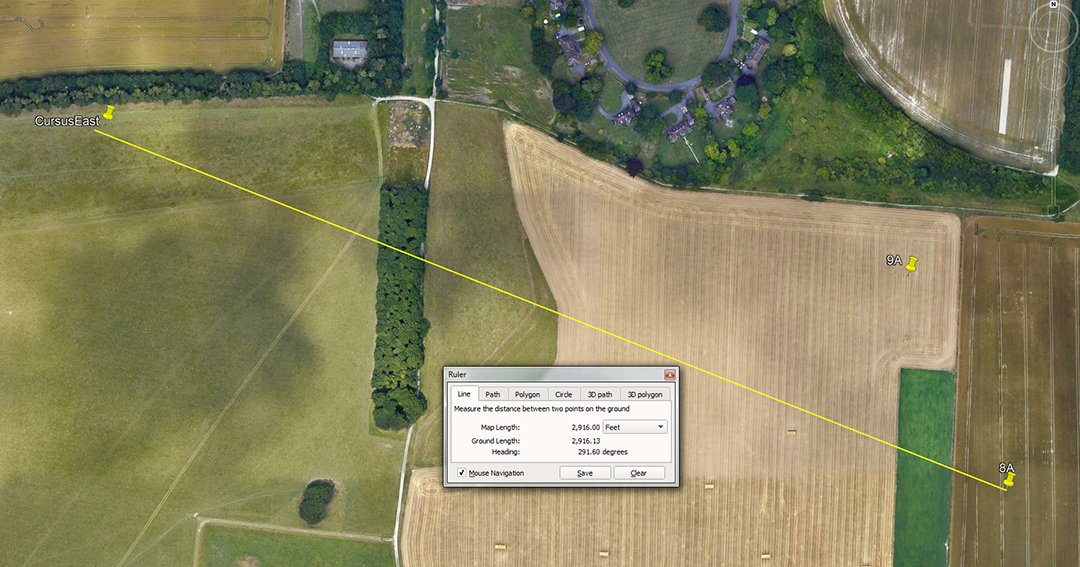The distance is 2916-feet and the angle is 291.6-degrees.

A mathematical progression based upon 2916 goes:

2916, 5832, 8748, 11664, 14580, 17496, 20412, 23328 ... 29160 ... 46656 ... 58320 ... 72900 ... 93312 ... 130636800.

This string allows for taking the 24883.2-miles of 5250-feet (130636800-feet) and then breaking it down by half, quarter, eighth, sixteenth, thirty-second, sixty-forth, etc., consistent with 320-640-1280, etc., parts within a 360 environment.

Therefore, 130636800 ÷ 32 = 4082400 ÷ 5250 = 777.6-miles ... or 360 ÷ 32 = 11.25-degrees ... or 24883.2 ÷ 32 = 777.6 miles.

8A TO ii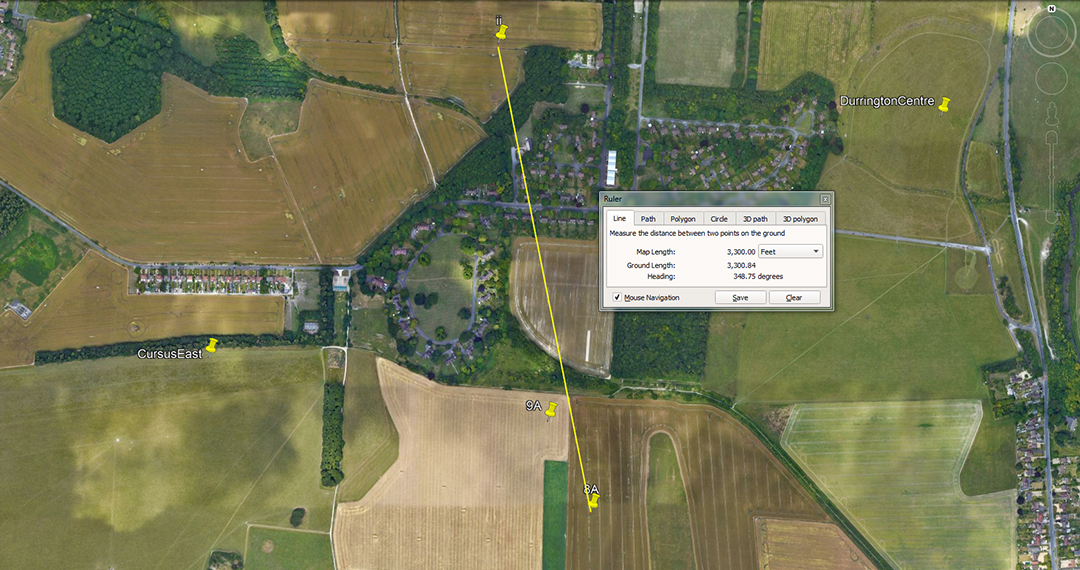The distance is 3300-feet and the return angle is 168.75-degrees.

A mathematical progression based upon 3300 goes:

3300, 6600, 9900, 13200, 16500, 19800 ... 26400 ... 29700 ... 36300 ... 52800 ... 59400 ... 363000, etc.

This relates to the furlong (660-feet), the English mile (5280-feet), the Scottish mile (5940-feet), the league (16500-feet), etc.

In the lineup we have 363000, which was 1-degree of equatorial arc (68.75-miles) in a world considered to be 130680000-feet or 24750-miles in circumference.

The angle is 168.75-degrees and a mathematical progression based on this goes:

168.75, 337.5, 506.25, 675, 843.75, 1012.5, 1181.25, 1350 ... 1687.5 ... 2025 ... 2700 ... 3543.75 ... 4050 ... 4725 ... 5400 ... 5906.25 ... 7087.5 ...

As can be seen, this string produces lunar numbers. There would be 21 periods of 16.875-days in a lunar year of 354.375-days.

ii TO IV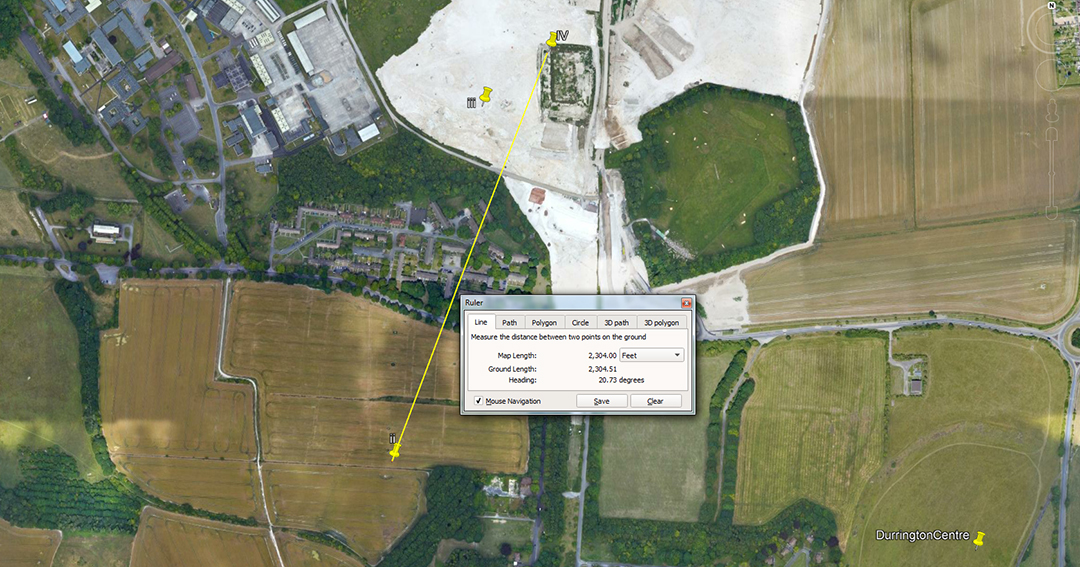The distance is 2304-feet and the angle is 20.736-degrees.

A mathematical progression based upon 2304 goes:

2304, 4608, 6912, 9216, 11520, 13824, 16128, 18432, 20736, 23040 ...34560 ... 41472 ... 57600 ... 62208 ... 124416 ... 248832.

This string relates to the 24883.2-mile equatorial circumference of which 345.6-miles would be 1/72nd or 5 degrees of arc. Alternatively 414.74-miles would be 1/60th of the equatorial circumference or 6-degrees of arc.

The angle of 20.736 is in homage to the largest Egyptian Royal cubit @ 20.736-inches, used as a mnemonic device for calculating the true size of the Earth. Therefore 20.736-inches (read as miles) X 1200 = 24883.2.

IV TO 11D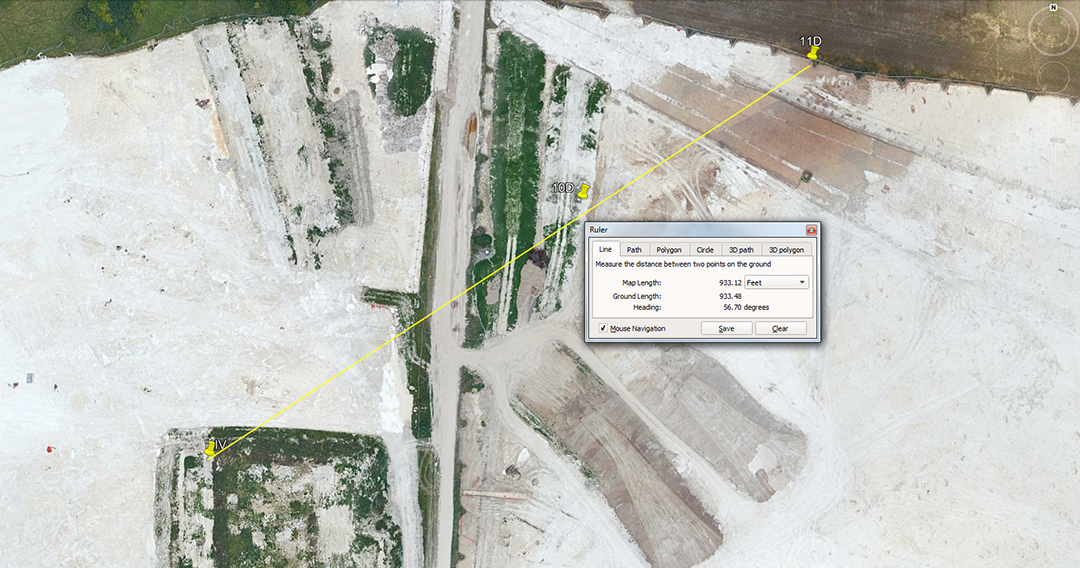The distance is 933.12-feet and the angle is 56.7-degrees. The distance would also have been read as 933 & 3/7ths-feet.

A mathematical progression based upon 933.12 goes:

933.12, 1866.24, 2799.36, 3732.48, 4665.6, 5598.72, 6531.84 ... 9331.2 ... 13063.68 ... 23328 ... 130636800.

This string increases to the value, in feet, for the equatorial circumference of the Earth configured at 130636800-feet or 24883.2-Greek miles of 5250-feet each.

Note that the 6531.84 value X 2 = 13063.68.

It's significant that the Babylonian/ Sumerian Talent weight was 933120-grains, encoding the value for 1/140th of the equatorial circumference of the Earth in Greek miles (thousands of years before there was a Greek civilisation).

Alternatively, a mathematical progression based upon 933 & 3/7ths feet would lead to the 130680000-feet reading (24750-miles of 5280-feet), wherein 933 & 3/7ths feet would be 1/140000ths of the Earth's equatorial circumference under that navigational assignment.

A mathematical progression based upon 56.7 goes:

56.7, 113.4, 170.1, 226.8, 283.5, 340.2, 396.9, 453.6, 510.3, 567 ... 680.4 ... 850.5 ... 907.2 ... 1134 ... 1417.5 ... 1701 ... 2551.5 ... 2835 ... 3402 ... 6804. .

This is lunar coding and navigational coding combined. The values 1701, 3402 & 6804 relate, of course, to 1/4th, 1/2 and the full duration, in days of the Lunar Nutation cycle.

The 2551.5 value provides the number of days the lunar year was monitored and followed within the lunisolar Sabbatical Calendar count (7.2 lunar years alongside 7 solar years) and 2835 relates to the full perimeter reading of the Khafre Pyramid of Egypt (a pyramid of the moon).

Values like 453.6 or 9072 relate to the Great Pyramid's navigational assignment.

11D TO 13DThe distance is 875-feet and the angle is 76.8-degrees. 880-feet would have featured in the tutorial.

A mathematical progression based upon 875 is covered for shafts 2A to 3A.

A progression based upon 76.8 goes:

76.8, 153.6, 230.40 307.2, 384, 460.8, 537.6, 614.4, 691.2, 768 ... 921.6 ... 1152 ... 12441.6 ... 24883.2.

This is just a variation on the 2304-code between stations ii to IV and relates to the equatorial circumference of the Earth.

A mathematical progression based upon 880 goes

880, 1760, 2640, 3520, 4400, 5280, 6160, 7040, 7920, 8800, 9680, 10560 ...52800.

Again, these values relate to the mile of 5280-feet.

13D TO 14D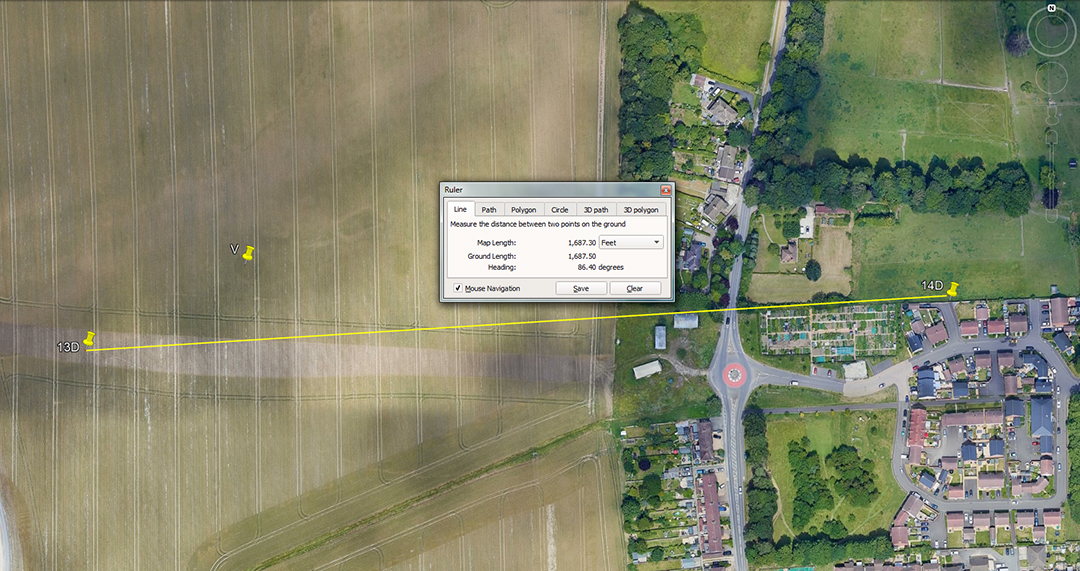The distance is 1687.5-feet and the angle is 86.4-degrees. The value 1701-feet would also have featured.

The distance code is found in relation to shaft 8A to ii.

A mathematical progression based upon 864 goes:

864, 1728, 2592, 3456, 4320, 5184, 6048, 6912, 7776, 8640 ... 10368 ... 15552 ... 20736 ... 25920 ... 31104 ... 36288 etc.

This string contains navigational codes like 6048 (1-minute of equatorial arc) or 362880 (1-degree of equatorial arc). Also, 25920 (the duration of the precession of the equinoxes in years) or 5184 (1/5th of the precession of the equinoxes in years) ... or 51.84 (the slope angle of the Great Pyramid ... etc). There's also 1728 (the number of cubic inches in a cubic foot.

For more information on the merits of this string see shafts 9A to ii.

14D TO DURRINGTON CENTRE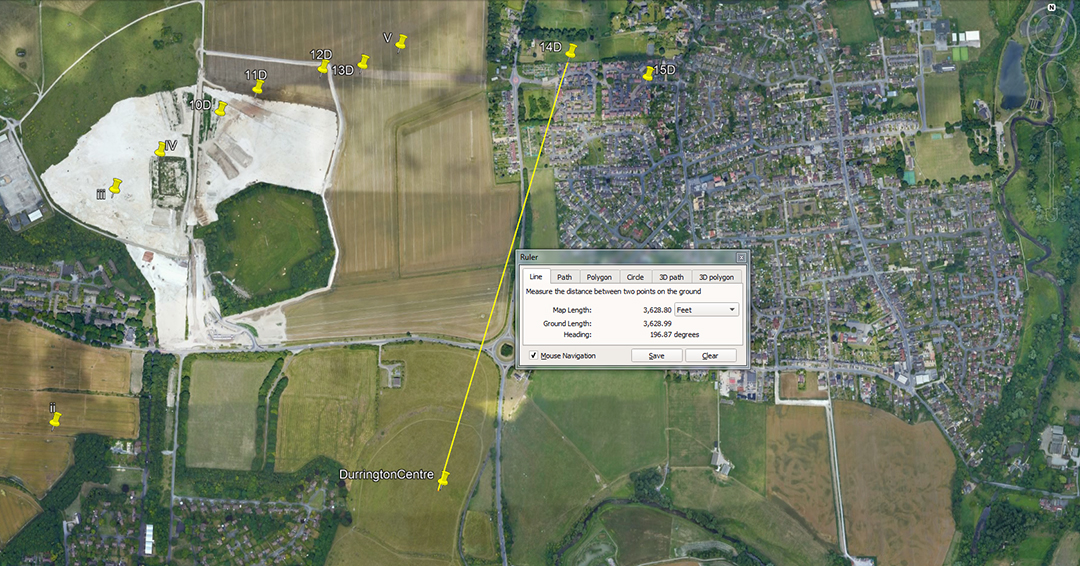The distance is 3628.8-feet and the angle is 196.875-degrees. The value 3600-feet would also have featured.

To recap, 362880-feet was 1-degree of arc in the 130636800-feet reading of the Earth's equatorial circumference.

The sum of 196.875-grains was the assay weight of 1 Egyptian Beqa of gold, adopted by both the Romans & Greeks for their gold standards of weight.

Continue